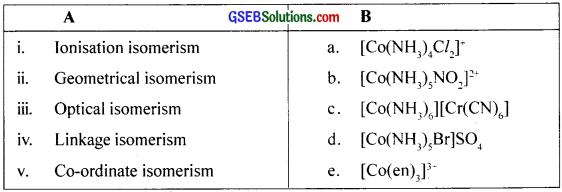# GSEB Solutions Class 12 Chemistry Chapter 9 Coordination Compounds

Gujarat Board GSEB Textbook Solutions Class 12 Chemistry Chapter 9 Coordination Compounds Textbook Questions and Answers, Additional Important Questions, Notes Pdf.

## Gujarat Board Textbook Solutions Class 12 Chemistry Chapter 9 Coordination Compounds

### GSEB Class 12 Chemistry Coordination Compounds InText Questions and Answers

Question 1.
Write the formulas for the following coordination compounds?

1. Tetraamminediaquacobalt(III) chloride
2. Potassium tetracyanidonickelate(II)
3. Tris(ethane-1,2-diamine) chromium(III) chloride
4. Amminebromidochloridonitrito-N-platinate(II)
5. Dichloridobis(ethane-1,2-diamine)platinum(IV) nitrate
6. Iron(III) hexacyanoferrate(II)

1. [Co(NH3)4(H2O)2]Cl3
2. K2[Ni(CN)4]
3. [Cr(en)3]Cl3
4. [Pt(NH3)BrCl(NO2)]
5. [PtCl2(en)2](NO3)2
6. Fe4[Fe(CN)6]3Question 2.
Write the IUPAC names of the following coordination compounds?

1. [CO(NH3)6]Cl3
2. [CO(NH3)5Cl]Cl2
3. K3[Fe(CN)6]
4. K3[Fe(C2O4)3]
5. K2[PdCl4]
6. [Pt(NH3)2Cl(NH2CH3)]Cl

1. Hexaamminecobalt(III) chloride
2. Pentaamminechloridocobalt(IIl) chloride
3. Potassium hexacyanidoferrate(III)
4. Potassium trioxalatoferrate(III)
6. Diamminechlorido(methanamine)platinum(II) chloride

Question 3.
Indicate the types of isomerism exhibited by the following complexes and draw the structures for these isomers:

1. K[Cr(H2O)2(C2O4)2]
2. [Co(en)3]Cl3
3. [CO(NH3)5(NO2)](NO3)2
4. [Pt(NH3)(H2O)Cl2]

1. Both geometrical (cis-, trans-) and optical isomers for one can exist.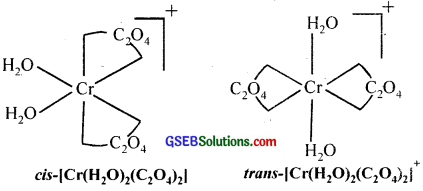2. Two optical isomers can exist.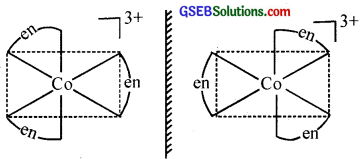3. There are 10 possible isomers. (Hint: There are geometrical, ionisation and linkage isomers possible).
The linkage isomers are given below
[CO(NH3)5NO2] (NO3)2 and [CO(NH3)5ONO] (NO3)2

4. Geometrical (cis-, trans-) isomers can exist.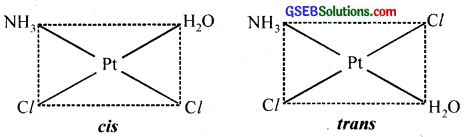Question 4.
Give evidence to show that [CO(NH3)5Cl]SO4 and [CO(NH3)5SO4]Cl are ionisation isomers?
Ionisation isomers when dissolved in water furnish different ions which can be tested.
[CO(NH3)5SO4]Cl + AgNO3 → [CO(NH3)5SO4]NO3 + AgCl ↓
White precipitate indicates that the isomer has Ck ion outside the coordination sphere.
[CO(NH3)5Cl]SO4 + BaCl2 → [CO(NH3)5Cl]Cl2 + BaSO4 ↓ ppt
White precipitate indicates the isomer has $$\mathrm{SO}_{4}^{2-}$$ ion outside the coordination sphere.

Question 5.
Explain on the basis of valence bond theory that [Ni(CN)4]2- ion with square planar structure is diamagnetic and the [NiCl4]2- ion with tetrahedral geometry is paramagnetic.
CN ion is a strong field ligand and when it approaches Ni2+ ion, it causes the pairing up of the two unpaired 3d electrons. It thus involves dsp2 hybridisation and has square planar structure. Due to the absence of unpaired electrons, [Ni(CN)4]2- is diamagnetic.

Cl is a weak field ligand and it is unable to pair up the two unpaired 3d electrons. It thus undergoes sp3 hybridisation and has tetrahedral geometry. Due to the presence of unpaired electrons, [NiCl4]2- is paramagnetic.

Question 6.
[NiCl4]2- is paramagnetic while [Ni(CO)4] is diamagnetic though both are tetrahedral. Why?
[NiCl4]2- has 2 unpaired electrons and is paramagnetic.
Like CN, Co is also a strong field ligand, same like Co, it causes pairing of electrons. No unpaired electrons left, hence it is diamagnetic.

Question 7.
[Fe(H2O)6]3+ is strongly paramagnetic whereas [Fe(CN)6]3- is weakly paramagnetic. Explain?
In presence of CN, (a strong ligand) the 3d electrons pair up leaving only one unpaired electron. The hybridisation is d2sp3 forming an inner orbital complex. In the presence of H2O, (a weak ligand), 3d electrons do not pair up. The hybridisation is sp3d2 forming an outer orbital complex containing five unpaired electrons, it is strongly paramagnetic.Question 8.
Explain [CONH3)6]3+ is an inner orbital complex whereas [Ni(NH3)6]2+ is an outer orbital complex?
In [CO(NH3)6]3+, CO is in a +3 oxidation state with the configuration 3d6. In the presence of NH3, 3d-electrons pair up leaving two d-orbitals empty. Hence, the hybridization is d2sp3 forming an inner orbital complex.

In [Ni(NH3)6]2+, Ni is in a +2 oxidation state with the configuration 3d6. In presence of NH3, the 3d-electrons do not pair up. The hybridization involved is sp3d2 forming outer orbital complex.

Question 9.
Predict the number of unpaired electrons in the square planar [Pt(CN)4]2- ion?
For square planar shape, the hybridisation is dsp2. Hence the unpaired electrons in 5d orbital pair up to make one ‘d’ orbital empty for dsp2 hybridisation. Thus there is no unpaired electron.

Question 10.
The hexaquamanganese(II) ion contains five unpaired electrons, while the hexacyanidomanganese (II) ion contains only one unpaired electron. Explain using Crystal Field Theory?
H2O is a weak field ligand and causes only small splitting of d orbitals. Consequently, the orbital splitting energy is not sufficiently large to force pairing of electrons in Mn (II) which has t2g3 eg2 configuration and thus hexaaquamanganese (II) ion contains five unpaired electrons. But CN ion is a strong field ligand and causes large splitting of d orbitals. Thus, Mn(II) has t2g5eg° configuration and thus contains only one unpaired electron in hexacyanidomanganese (II) ion.Question 11.
Calculate the overall complex dissociation equilibrium constant for the [Cu(NH3)2+4] ion, given that β4 for this complex is 2.1 × 1013.
The overall dissociation constant is the reciprocal of overall stability constant i.e. $$\frac { 1 }{ \beta _{ 4 } }$$ = 4.7 × 10-14.

### GSEB Class 12 Chemistry Coordination Compounds Text Book Questions and Answers

Question 1.
Explain the bonding in coordination compounds in terms of Werner’s postulates?
The coordination compounds were known since 18th century but no satisfactory theory was available to explain the observed properties of these compounds. In a series of compounds of cobalt (III) chloride with ammonia it was found that some of the chloride ions are precipitated as AgCl on adding excess of silver nitrate solution in cold and some remained in solution.

For example, when the coordination compound, COCl3.6NH3 is treated with excess of AgNO3, all the three chlorine atoms arc precipitated as AgCl whereas when COCl3.5NH3 is used onÍy two chlorine atoms are precipitated.

This means that in the first compound all the three chlorine atoms behave as chloride ions whereas in the second case only two chlorine atoms behave as chloride ions. Similarly, the number of chloride ions which are precipitated in the case of COCl3.4NH3 and COCl3.3NH3 is found to be I and none respectively. These observations support the structural formula as described below.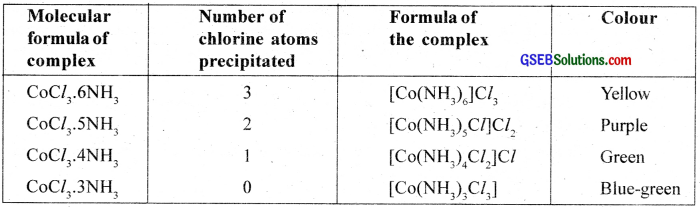Alfred Werner in 1893 put forward his concept of auxiliary (secondary) valency advancing a correct explanation for the characteristics of the coordination compounds. The fundamental postulates of Werner’s theory are summarised below.

1. Metals possess two types of valencies – primary and secondary valencies. The primary valency is ion is able while the secondary valency is non – ion ionasible.
2. Every metal atom or ion lias a fixed number of secondary valencies equal to its coordination number.
3. The primary valencies are satisfied by negative ions and the secondary valencies by negative ions or neutral groups (ligands).
4. The primary valencies are non – directional. The ligands satisfying the secondary valencies are always directed towards fixed positions in space, giving a definite geometry to the complex.

In modem formulations, the spatial arrangements are called coordination polyhedra. The species within the square bracket are called coordination complexes and the ions outside the square bracket are called counter ions.

On the basis of Werner’s theory, the complex COCl3.6NH3 or [Co(NH3)6]Cl3. [hexaarnminecobalt (III) chloride] can be represented as shown in the figure.

Here three dotted lines indicate the three primary valencies (oxidation state) of cobalt; while the six thick lines indicate its secondary valencies (coordination number).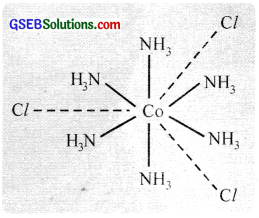Differences between a double salt and a complex:
Both double salts and complexes are formed by the combination of two or more stable compounds in a stoichiometric ratio. However, they differ in the fact that double salts such as carriallite (KCl.MgCl2.6H2O). Mohr’s salt (FeSO4.(NH4)2,SO4.6H2O), alums etc. dissociate into simple ions completely when dissolved in water. However, complex ions such as [Fe(CN)6]3- of K3[Fe(CN)6] does not dissociate into Fe3+ and CN ions.Question 2.
FeSO4 solution mixed with (NH4)2SO4 solution in 1:1 molar ratio gives the test of Fe2+ ion but CuSO4 solution mixed with aqueous ammonia in 1:4 molar ratio does not give the test of Cu2+ ion. Explain why?
FeSO4 when mixed with (NH4)2SO4 in a 1:1 ratio forms a double salt, FeSO4.(NH4)2SO4.6H2O (Mohr’s salt) ionises in solution to give Fe2+ ions. Hence, it gives the tests of Fe2+ ions. But CuSO4 solution when mixed with aqueous ammonia in 1:4 ratio forms a complex [Cu(NH3)4]SO4. The complexion [Cu(NH3)4]2+ does not ionise further to give Cu2+ ions and does not give the tests of Cu2+ ion.

Question 3.
Explain with two examples each of the following: coordination entity, ligand, coordination number, coordination polyhedron, homoleptic and heteroleptic?
1. Central ion or centre of coordination:
The cation to which one or more neutral molecules or anions are attached is called the centre of coordination or central ion. In the complexes Ni(NH3)6]2+ and [Fe(CN)6]3-, Ni2+ and Fe3+ respectively are the central ions. The central atom/ions are also referred to as Lewis acids.

2. Ligand:
An atom or group of atoms which binds to the central atom through the lone pair of electrons present in its donor atom is called a ligand. Depending on the number of pairs donated by different atoms, ligands can be classified as unidentate (one), bidentate (two), and polydentate (more than two) ligands.
Monoclentute ligande provides one electron pair per molecule or ion. The species NH3, H2O, CO, Cl, F, OH etc are of this type.

Bidentate ligands furnish two electron pairs per molecule or ion. i.e., they are bonded to a metal ion through two sites or atoms.

e.g. Ethylenediamine (en)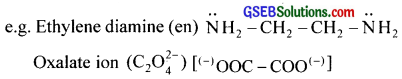Polydentate ligand supplies several pairs of electrons i.e., they have several donor sites. Thus EDTA (Ethylenediaminetetraacetic acid) is hexadentate with six donor groups, namely two nitrogen and four oxygen atoms from four carboxylic acid groups.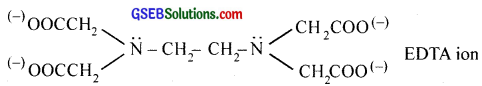3. Ambidentate ligands:
Are monodentate ligands which can ligate through two different atoms present in it. For example, NO2 ion can coordinate either through nitrogen or through oxygen to a central metal atom/ion.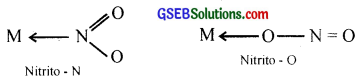Similarly, SCN ion can coordinate through sulphur or nitrogen atom. But ambidentate ligands are not bidentate ligands because they cannot coordinate to the central metal ion simultaneously from two atoms.

4. Chelating ligand:
When a bidentate or polydentate Iiand binds to a central atom and forms a ring like structure called ‘chelate’, the ligand is known as chelating ligand. The chelate rings formed between Cu2+ and ethylene diamine is given below.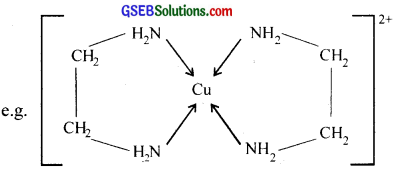The chelating ligands form more stable co-ordination compounds than the monodentate analogs.

5. Coordination sphere:
The central atom along with ligands surrounding it is written in a square bracket, [] is called coordination sphere. The atoms, ions or molecules in this sphere are non-ionizable. The ionisable groups are written outside the bracket and are called counter ions.
e.g. In K4[Fe(CN)6], the coordination sphere is [Fe(CN)6]4- and the K+ is the counter ion.

6. Coordination number:
The total number of ligands surrounding the central atom in the coordination sphere is the coordination number. e.g. In [Ni(NH3)6]Cl2, Ni2+ forms six bonds with 6 NH3 molecules, it has a coordination number six. In [Cu(NH3)4]2+, the coordination number of Cu is four.

7. Charge on complex:
The sum of oxidation numbers of all the atoms/molecules or ions constituting the coordination sphere is the charge on the complex.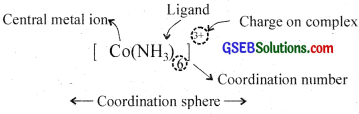8. Coordination polyhedron:
The spatial arrangement of the ligand atoms which are directly attached to the central atom/ion defines a coordination polyhedron about the central atom. Tetrahedral, square planar, octahedral, square pyramidal, and trigonal bipyramidal are common shapes of coordination polyhedra.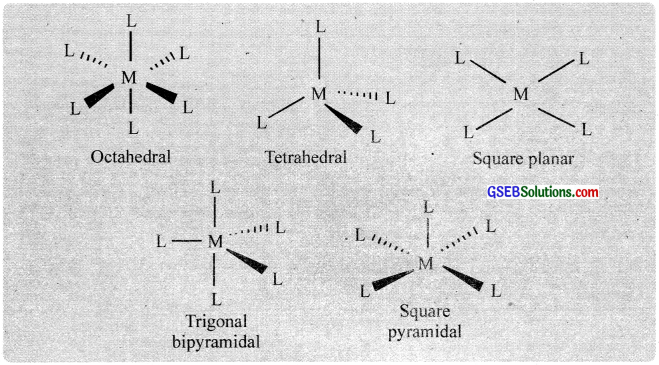9. Homoleptic and heteroleptic complexes:
Complexes in which a metal is bound to only one kiñd of ligands are known as homoleptic complexes. e.g. [CO(NH3)4Cl2]+ Complexes in which a metal is bound to more than one kind of ligands are known as heteroleptic complexes.
e.g. [CO(NH3)4Cl2]+.Question 4.
What is meant by unidentate, didentate, and ambidentate ligands? Give two examples for each?
1. Ligand:
An atom or group of atoms which binds to the central atom through the lone pair of electrons present in its donor atom is called a ligand.

Depending on the number of pairs donated by different atoms, ligands can be classified as unidentate (one), bidentate (two), and polydentate (more than two) ligands.

Monoclentute igandc provides one electron pair per molecule or ion. The species NH3, H2O, CO, Cl, F, OH etc are of this type.

Bideniate ligands furnish two electron pairs per molecule or ion. i.e., they are bonded to a metal ion through two sites or atoms.

e.g. Ethylenediamine (en)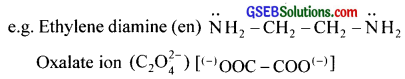Polydentate ligand supplies several pairs of electrons i.e., they have several donor sites. Thus EDTA (Ethylenediaminetetraacetic acid) is hexadentate with six donor groups, namely two nitrogen and four oxygen atoms from four carboxylic acid groups.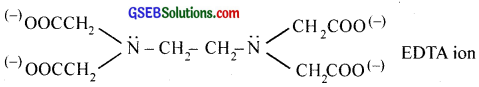2. Ambidentate ligands:
Are monodentate ligands which can ligate through two different atoms present in it. For example, NO2 ion can coordinate either through nitrogen or through oxygen to a central metal atom/ion.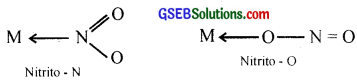Similarly, SCN ion can coordinate through sulphur or nitrogen atom. But ambidentate ligands are not bidentate ligands because they cannot coordinate to the central metal ion simultaneously from two atoms.

Question 5.
Specify the oxidation numbers of the metals in the following coordination entities:

1. [CO(H2O)(CN)(en)2]2+
2. [PtCl4]2-
3. [PtCl4]2-
4. K3[Fe(CN)6)]
5. [Cr(NH3)3Cl3]

1. +3
2. +3
3. +2
4. +3
5. +3Question 6.
Using IUPAC norms write the formulas for the following: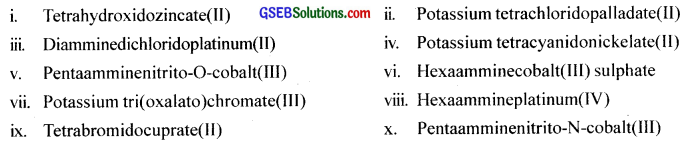1. [Zn(OH)4]2-
2. K2[PdCl4]
3. [Pt(NH3)2Cl2]
4. K2[Ni(CN)4]
5. [CO(NH3)5(ONO)]2+
6. [CO(NH3)6]2(SO4)3
7. K3[Cr(C2O4)3]
8. [Pt(NH3)6]4+
9. [CuBr4]2-
10. [CO(NH3)5(NO2)]2+

Question 7.
Using IUPAC norms write the systematic names of the following:

1. [CO(NH3)6]Cl3
2. [Pt(NH3)2Cl(NH2CH3)]Cl
3. [Ti(H2O)6]3+
4. [CO(NH3)4Cl(NO2)]Cl
5. [Mn(H2O)6]2+
6. [NiCl4]2-
7. [Ni(NH3)6]Cl2
8. [CO(en)3]3+
9. [Ni(CO)4]

1. Hexaamminecobalt (III) chloride
2. Diaamminechlorido (methenamine) platinum (II) chloride
3. Hexaaquatitanium (III) ion
4. Tetraamminechloridonitrito-N-cobalt (III) chloride
5. Hexaaquamanganese (II) ion
6. Tetrachloridonickelate (II) ion
7. Hexaamminenickel (II) chloride
8. Tris(ethylenediammine) cobalt (III) ion
9. Tetracarbonylnickel (0)Question 8.
List various types of isomerism possible for coordination compounds, giving an example of each?
The compounds which have the same chemical composition but have a different structural arrangement of their atoms are called isomers and the phenomenon is called isomerism. Coordination compounds exhibit structural isomerism and stereoisomerism. Each of these is further subdivided as described below.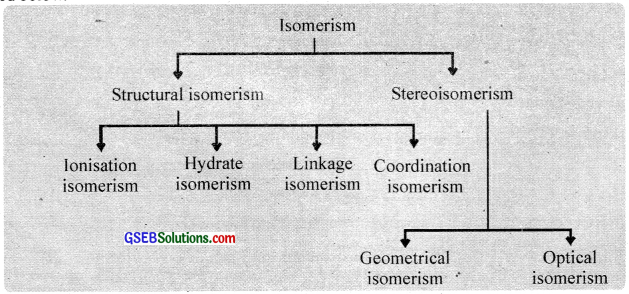Structural isomerism:
This isomerism arises due to the difference in structures of coordination compounds. This is of the following types.

1. Ionisation isomerism:
This arises when the coordination compounds with the same molecular formula give different ions in solution. For example, violet coloured pentaamminehromidocobalt (III) sulphate is an ionisation isomer of red-coloured pcntaamrninesulphatocobalt (III) bromide.
[CO(NH3)5Br] SO4 (Violet) pentaarnminebromidocobalt (III) sulphate
[CO(NH3)5SO4]Br (Red) pentaamminesulphatocobalt (III) bromide

It arises in complexes containing monodentate ligands with more than one donor atom. e.g., NO2 may be bonded to a metal through nitrogen(nitrito-N) or through oxygen (- O – N = O) (nitrito – O). Such ligands arc also known as ambidentate ligands.
For example.
[CO(NH3)5NO2]Cl2 pentaamrninenitrito – N – cobalt (III) chloride (Yellow)
[CO(NH3)5ONO]Cl2, pentaamrninenitrito – O – cobalt (III) chloride (Red)
Another common ambidentate ligand is CNS
e.g.[Cr(H2O)5SCN]2+ and [Cr(H2O)5NCS]2+

3. Coordination isomerism:
This type of isomerism is possible when both positive and negative ions of a salt arc complexions. it involves the exchange of ligands between complex cation and complex anion i.e., between the coordination spheres in a compound. For example,
[Cr(NH3)6] [CO(CN)6 hexaamminechromiurn (III) hexacyanidocobaltate (III)
[CO(NH3)6 [Cr(CN)6 hexaamm inecobalt (III) hexacyan idochromate (III)

4. Hydrate isomerism:
It arises in complexes due to a different number of water molecules present in the coordination spheres. For example,
[Cr(H2O)6Cl3 (violet) hexaaquachromium (III) chloride
[Cr(H2O)5Cl2.H2O (grey-green) pentaaquachloridochrornium (III) chloride monohydrate

Stereoisomerism:
When two compounds contain the same ligands coordinated to the same central ion, but the special arrangement of ligands around the central atom is different, the two compounds are said to be stereoisomers and the type of isomerism is called stereoisomerism. It is of two types:

1. Geometrical isomerism or cis-trans isomerism and
2. Optical isomerism.

1. Geometrical isomerism:
Geometrical isomerism arises when ligands occupy different positions around the central ion. The ligands may occupy positions either adjacent to one another or opposite to one another. These are referred to as cis – form and transform respectively. This type of isomerism is, therefore, referred to as cis-trans isomerism. Geometrical isomerism of compounds with coordination numbers 4 and 6 is most important.

(a) Square planar complexes:
(coordination no. 4) with formula Ma2b2 and Ma2bc show cis-trans isomerism. e.g. [Pt(NH3)2Cl2]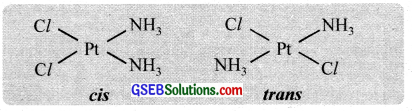(b) Octahedral complexes (coordination no. 6) with the formula Ma4b2 and Ma4b2 and Ma4bc, show cis-trans isomerism.
e.g. [CO(NH3)4Cl2]+, [CO(en)2Cl2]+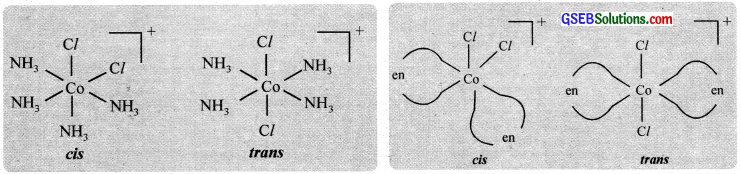Another type of geometrical isomerism occurs in octahedral complexes of the type [Ma3b3] like [CO(NO2)3(NH3)3. If each trio of donor atoms occupy adjacent positions at the corners of an octahedral face, we have the facial (fac) isomer and when the positions are around the meridian of the octahedron, we get the meridional (mer) isomer.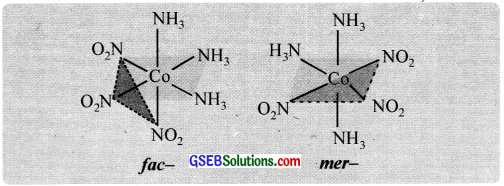2. Optical isomerism:
Optical activity is the ability of a compound to rotate the plane of plane polarised light. An optically active compound can be obtained in d – and i – isomeric forms (enantiomers), the d – isomer will rotate the plane of polarized light to the right (dextro) and the i – isomer to the left (laevo).

Optical isomerism is shown by the chelates like [Co(en)3]Br3. This compound has no plane of symmetry and it gives rise to non – superimposable mirror images. The optical isomers have identical, physical and chemical properties. They differ only in the direction in which they rotate the plane of plane polarised light.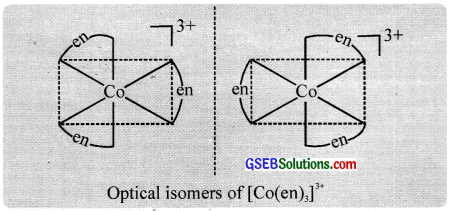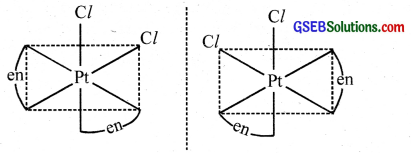In the coordinate entity of the type [PtCl2(en)2]2+, only the cis isomer shows optical isomerism.Question 9.
How many geometrical isomers are possible in the following coordination entities?

1. [Cr(C2O4)3]3-
2. [CO(NH3)3Cl3]

1. [Cr(C2O4)3]3- – Nil
2. [CO(NH3)3Cl3] – Two (fac- and mer-)

Question 10.
Draw the structures of optical isomers of:

1. [Cr(C2O4)3]3-
2. [PtCl2(en)2]2+
3. [Cr(NH3)2Cl2(en)]+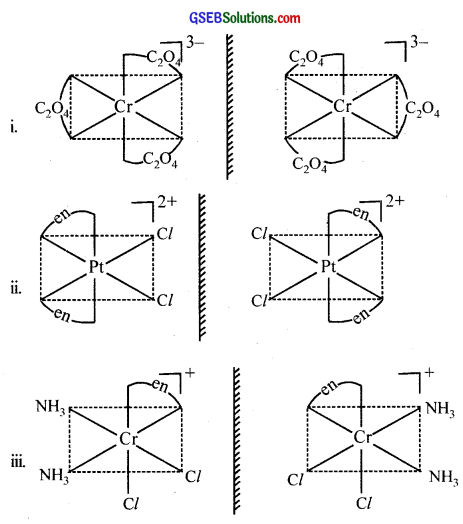Question 11.
Draw all the isomers (geometrical and optical) of:

1. [COCl2(en)2]+
2. [CO(NH3)Cl(en)2]2+
3. [CO(NH3)2Cl2(en)]+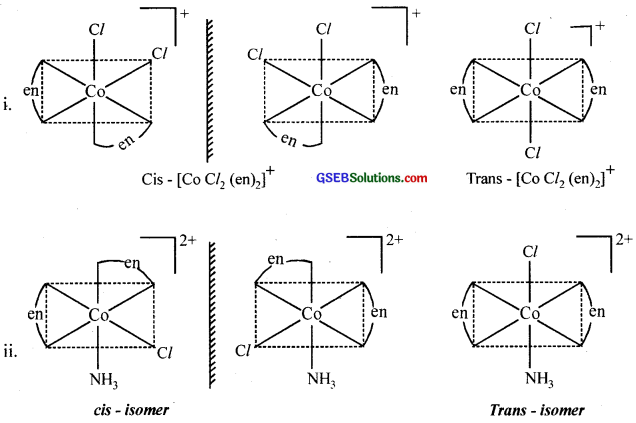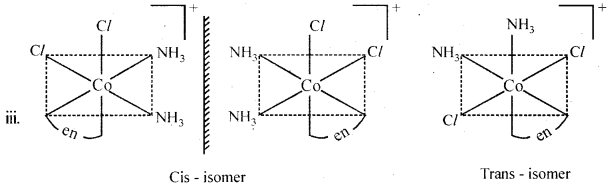Question 12.
Write all the geometrical isomers of [Pt(NH3)(Br)(Cl)(py)] and how many of these will exhibit optical isomerism?
Three isomers are possible.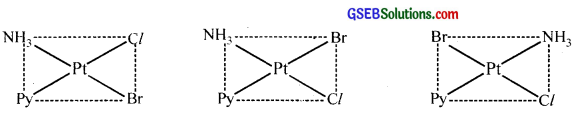These isomers do not show optical isomerism (square planar complexes do not show optical isomerism).Question 13.
Aqueous copper sulphate solution (blue in colour) gives:

1. A green precipitate with aqueous potassium fluoride and
2. A bright green solution with aqueous potassium chloride. Explain these experimental results?

Aqueous CuSO4 solution exists as [Cu(H2O)SO4 which has blue colour due to [Cu(H2O)4]2+ ions.

1. When KF is added, the weak H2O ligands are replaced by F ligands, forming [CuF4]2- ions which is a green precipitate.
[CU(H2O)4]2+ + 4F → [CuF4]2- + 4H2O

2. When KCl is added, Cl ligands replace the weak H2O ligands forming [CuCl4]2- ions which has bright green colour.
[Cu(H2O)4]2+ + 4Cl → [CuCl4]2- + 4H2O

Question 14.
What is the coordination entity formed when an excess aqueous KCN is added to an aqueous solution of copper sulphate? Why is it that no precipitate of copper sulphide is obtained when H2S(g) is passed through this solution?
[Cu(H2O)4]2+ + 4CN → [Cu(CN)4]2- + 4H2O
As CN is a strong ligand, it forms a highly stable complex with Cu2+ ion. On passing H2S, free Cu2+ ions are not available to form the precipitate of CuS2.

Question 15.
Discuss the nature of bonding in the following coordination entities on the basis of valence bond theory:

1. [Fe(CN)6]4-
2. [FeF6]3-
3. [CO(C2O4)3]3-
4. [COF6]3-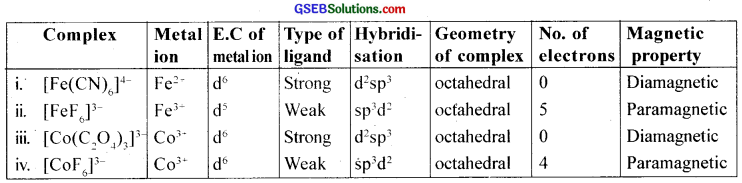Question 16.
Draw a figure to show the splitting of d orbitals in an octahedral crystal field?
Werner’s theory is incapable of explaining:

1. Why only certain metals form complexes.
2. The geometry and shape of complexes and
3. The spectral and magnetic properties of metal complexes.

Thus, Linus Pauling (1931) extended valence bond theory to the study of complexes.

Pauling’s valence bond theory (VBT):
The main points of valence bond theory are:

1. The central metal ion in the complex makes available an adequate number of vacant orbitais for the formation of coordinate bonds with the ligands.
2. The number of empty orbitais made available is equal to the coordination number of the central metal ion.
3. The Adequate number of vacant orbitais (s, p or d) of the central metal ion hybridise to give an equal number of vacant hybrid orbitais, which are directed towards the ligand positions according to the geometry of the complex.
4. The empty hybrid orbitais of the metal ion overlap with the fully filled orbitais of the ligand, forming the ligand-metal coordinate bond.
5. The outer orbital (high spin) or inner orbital (low spin) complexes are formed depending upon whether the d orbitaIs of the outer shell or inner shell are used for hybridisation.

Types of hybridisation and geometries of complexes: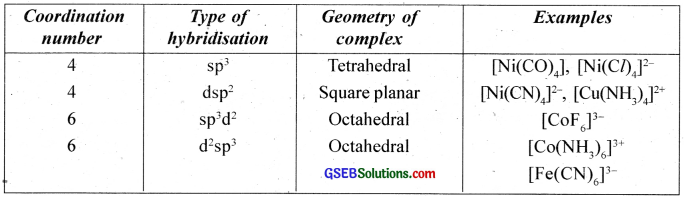Ligands can be classified into two types:-

(a) Strong field ligands cause maximum pairing of electrons.
(b) Weak field ligands which do not cause electron pairing.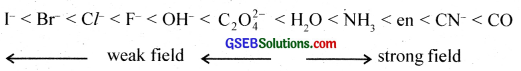Illustration:
(i) Hexaamminecobalt (III) ion, [CO(NH3)6]3+
In this complex, cobalt is in a +3 oxidation state. The outer electronic configuration of the CO3+ ion is 3d6. NH3 is a strong field ligand and hence the electrons in 3d orbitaIs are forced to pair up against Hund’s rule and make available two 3d empty orbitais.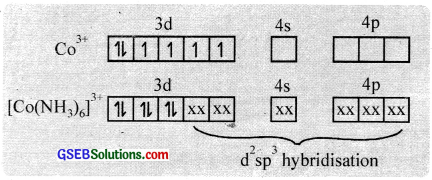Two 3d, one 4s and three 4p orbitaIs hybridise to give six vacant d2sp3 hybrid orbitais which accept electron pairs from six NH3 to form [CO(NH3)6]3+. The resulting complex is a low spin (diamagnetic – no unpaired electrons) inner orbital complex with an octahedral shape.

(ii) Hexafluoridocobaltate (III) ion, [COF6]3-
In this complex cobalt is in a +3 state. The outer electronic configuration of CO3+ is 3d6. Since F is a weak field ligand, it is unable to pair up the unpaired electrons in 3d orbitais. Therefore, one 4s, three 4p and two 4d orbitais hybridise to give six vacant sp3d2 hybrid orbitaIs which accept electron pairs from six F to form [COF6]3+.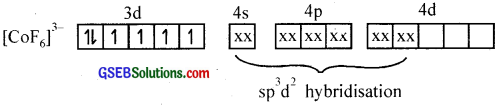The resulting complex is a high spin (paramagnetic – has four unpaired 3d electrons) outer orbital complex with an octahedral shape.

(iii) Tetracyanidonickelate (II) ion, INI (CN)4)2-
In this complex, Ni is in a +2 state. The electronic configuration of Ni2+ ion is 3d8.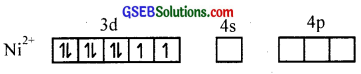Since, CN ion is a strong field ligand, the two unpaired electrons are forced to pair up against Hund’s rule and make available a vacant 3d orbital. One 3d, one 4s, and two 4p orbitais hybridise to give four dsp2 hybrid orbitais which accept electron pairs from four CN ions to form [Ni(CN)4]2- ion.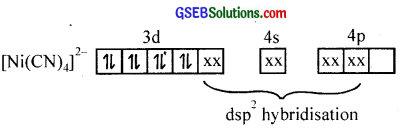The resulting complex is a low spin (diamagnetic – no unpaired electrons) inner orbital complex with square planar geometry.

(iv) Tetrachioridonickelate (II) ion, [NiCl4]2-
In this complex, Ni is in a Ni2+ state. The electronic configuration of Ni2+ is 3d8. Since Cl is a weak field ligand it is unable to pair up the unpaired electrons in 3d orbitaIs. Therefore, 4s and 4p orbitais hybridise to form four vacant sp3 hybrid orbitais which accept electron pairs from four Cl ions to form [NiCl4]2- ion.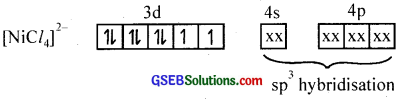The resulting complex is a high spin (paramagnetic – two unpaired electrons) outer orbital complex with tetrahedral geometry.

(v) Tetracarbonylnickel (O), [Ni(CO)4]
Here nickel ¡s present in a zero oxidation state. Ni has 3d84s2 electronic configuration. Since CO is a strong field ligand, the 4s electrons are forced to pair up against the Aufbau principle to make the 4s orbitais empty.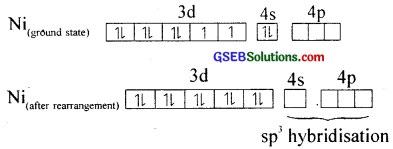The empty 4s and three 4p orbitais undergo sp3 hybridisation to form four equivalent hybrid orbitals which accept electron pairs from four CO and form Ni(CO)4.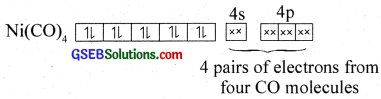Thus, nickel tetracarhonyl is a low spill (diamagnetic – no unpaired electron) outer orbital complex with tetrahedral geometry.

Crystal field theory:
The drawbacks of the valence bond theory of coordination compounds are to a great extent, removed by the crycial field theory proposed by H. Bethe and Van Vleck (1930). The crystal field theory regards the metal ion as situated in an electrostatic field caused by surrounding molecules or ions.

The five d – orbitais in an isolated gaseous metal atom/ion have the same energy i.e., they are degenerate orbitais. When the ligands approach the central atom, the degeneracy of the d – orbitais is lifted. It results in the splitting of the d – orbital energies. This conversion of five degenerate d-orbitais of the metal ion into different sets of orbitals having different energies in the presence of the electrical field of ligands is called crvstalfìeld splitting. The pattern of splitting depends upon the nature of the crystal field.

Splitting in the octahedral field:
As the ligands approach the central atom, energy of the d – orbitais increases, and then electrons in the d$$x^{ 2 }-y^{ 2 }$$ and d$$z^{ 2 }$$ orbitaIs having their lobes along the axes are repelled by the ligand electron pairs more than the electrons in the dxy, dxz and dyz orbitaIs, which have lobes between the axes.

Thus the five d – orbitais in an octahedral field are split into two sets d$$z^{ 2 }$$ and d$$x^{ 2 }-y^{ 2 }$$ (designated as ‘eg’) and dxy, dxz and dyz (designated as ‘t2g’). The energy separation known as crystal field splitting energy between eg and t2g orbitals is denoted by the symbol ∆0 (the subscript ‘o’ is octahedral).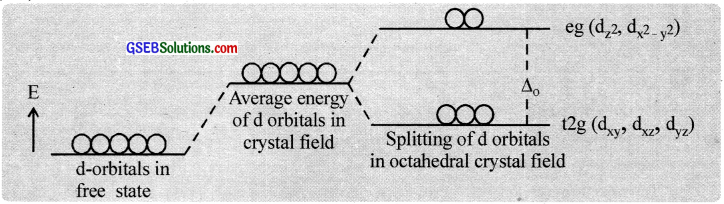In d1 coordination complexes. the single d-electron occupies one of the lower t2g orbitals. In d2 and d3 coordination entities, the d – electrons occupy the t2g orbitais singly in accordance with Hund’s rule. For d4 ions, two possible patterns of electron distribution arise. The electron can enter either the t2g or eg levels. The actual configuration is determined by the relative energies of ∆0 and P (P-pairing energy).

If ∆0 < P, we have the so-called weak field. high spin situation and the fourth electron enters one of the eg orbitais and if ∆0 > P, we have the strong field, low spin situation and the pairing will occur in the t2g level.

Splitting in the tetrahedral field:
In the tetrahedral field, the ligands approach the metal ion through a position in between the axes and the d – orbitais along the cartesian axes (d$$x^{ 2 }-y^{ 2 }$$ and d$$z^{ 2 }$$) are less repelled than dxy, dxz and dyz orbitaIs. Therefore, the eg orbitais have low energy and t2g orbitais have relatively high energy.

Thus in a tetrahedral field the d – orbital splitting is inverted. But the field splitting and splitting energies are smaller as compared to the octahedral field splitting. The splitting energy ∆t = $$\frac{-4}{9}$$ ∆0 (‘t’ for tetrahedral). In a tetrahedral field, low spin complexes are rarely observed.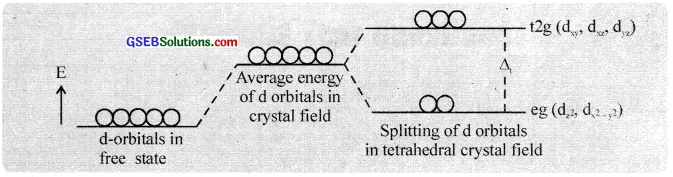Question 17.
What is spectrochemical series? Explain the difference between a weak field ligand and a strong field ligand?
The arrangement of ligands in the order of their increasing field strength (CFSE) is called the spectrochemical series. The ligands with small CFSE are called weak field ligands and those with large CFSE are called strong field ligands.

Question 18.
What is crystal field splitting energy? How does the magnitude of ∆0 decide the actual configuration of d orbitals in a coordination entity?
The difference in energy between the two sets of orbitals is called crystal field splitting energy, ∆0.
If ∆0 < P(pairing energy), the fourth electron in octahedral field enters into eg orbitals and form high spin complexes and if ∆0 > P, the fourth electron pairs up in the t2g orbitals and form low spin complexes.

Question 19.
[Cr(NH3)6]3+ is paramagnetic while [Ni(CN)4]2- is diamagnetic. Explain why?
In [Cr(NH3)6]3+, the central metal ion Cr3+ has 3d3 electronic configuration and undergoes d2sp3 hybridisation. Due to the presence of three unpaired electrons, it is paramagnetic.

In [Ni(CN)4]2-, the central metal ion Ni2+ has 3d8 electronic configuration. Since CN is a strong field ligand, it can pair up electrons in d subshell and makes available a vacant d orbital and undergoes dsp2 hybridisation. Due to the absence of unpaired electrons, [Ni(CN)4]2- is diamagnetic.

Question 20.
A solution of [Ni(H2O)6]2+ is green but a solution of [Ni(CN)4]2- is colourless. Explain?
In [Ni(H2O)6]2+, Ni2+ has 3d8 configuration. Since H2O is a weak field ligand, the unpaired electrons do not pair up. Thus there is unpaired d electrons and the complex is coloured. In [Ni(CN)4]2-, Ni2+ has 3d8 configuration. Since CN is a strong field ligand, the two unpaired electrons in the 3d orbitals pair up. Because of the absence of unpaired electrons, it is colourless.

Question 21.
[Fe(CN)6]4- and [Fe(H2O)6]2+ are of different colours in dilute solutions. Why?
In both complexes, Fe is in +2 state with 3d6 configuration. In presence of weak field H2O, the unpaired electrons do not pair up. But in presence of strong CN ligand, the unpaired electrons pair up leaving no unpaired electrons. Because of the difference in the number of unpaired electrons, they show different colours.Question 22.
Discuss the nature of bonding in metal carbonyls?
The M – C bond in metal carbonyl has σ as well as π character. The metal carbon σ bond formed by the donation of lone pair of electrons on the carbonyl carbon into a vacant orbital of the metal is as shown below. The metal-carbon π bond is formed by the donation of a pair of electrons from a filled d-orbital of metal into the vacant antibonding pi-molecular orbital (π) ofcarbon monoxide.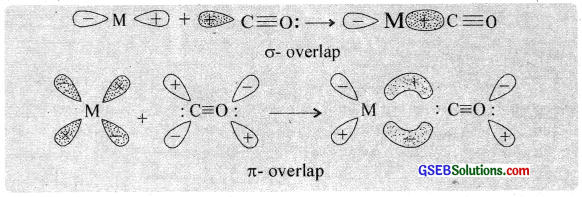Question 23.
Give the oxidation state, d orbital occupation and coordination number of the central metal ion in the following complexes:

1. K3[CO(C2O4)3]
2. cis-[Cr(en)2Cl2]Cl
3. (NH4)2[CoF4]
4. [Mn(H2O)6]SO4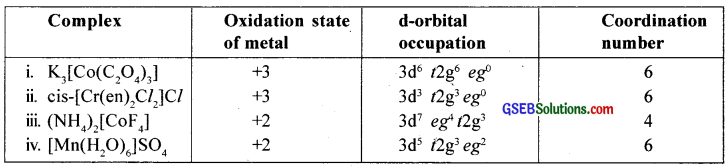Question 24.
Write down the IUPAC name for each of the following complexes and indicate the oxidation state, electronic configuration and coordination number. Also give stereochemistry and magnetic moment of the complex:

1. K[Cr(H2O)2(C2O4)2].3H2O
2. [Co(NH3)5Cl]Cl2
3. [CrCl3(py)3]
4. Cs[FeCl4]
5. K4[Mn(CN)6]

Oxidation state of Cr = + 3
Electronic configuration of Cr3+ = 3d3 = t2g3 eg0
Coordination number = 6
Shape = octahedral
Magnetic moment = $$\sqrt{n(n+2)}$$ BM = $$\sqrt{3(5)}$$ = 3.87 BM

2. Pentaamminechloridocobalt (III) chloride
Oxidation state of Co = +3
Electronic configuration of Co3+ = 3d6 = t2g6eg0
Coordination number = 6
Shape = octahedral
Magnetic moment = 0

3. Trichloridotripyridine chromium (III)
Oxidation state of Cr = +3
Electronic configuration of Cr3+ = 3d3 = t2g3eg0
Coordination number = 6
Shape = octahedral
Magnetic moment = $$\sqrt{n(n+2)}$$ BM = $$\sqrt{3(5)}$$ = 3.87 BM

4. Caesium tetrachloridoferrate (II)
Oxidation state of Fe = +3
Electronic configuration of Fe3+ = 3d5 = eg2 t2g3
Coordination number = 4
Shape = tetrahedral
Magnetic moment = $$\sqrt{n(n+2)}$$ BM = $$\sqrt{1(3)}$$ BM = 5.92 BM

5. Potassium hexacyanidomanganate (II)
Oxidation state of Mn = + 2
Electronic configuration of Mn2+ = 3d5 = t2g5 eg0?
Coordination number = 6
Shape = octahedral
Magnetic moment = $$\sqrt{n(n+2)}$$ BM = $$\sqrt{1(3)}$$ BM = 1.73 BMQuestion 25.
What is meant by stability of a coordination compound in solution? State the factors which govern stability of complexes?
The reaction between a metal ion and its ligands may be considered as a Lewis acid-base reaction. 1f the interaction is strong, the complex formed would be more stable from the thermodynamic point of view. The reaction between a metal ion and its ligands is represented in the following equilibrium.

Ma+ + nLX- ⇄ [MLn]b+ whcre a+, x- and b+ are the charges on the metal, ligand and complex respectively. [(a+) + n(x -) b + 1. The stability constant K of the complex can be defined as K = $$\frac { [ML_{ n }]^{ b+ } }{ [M^{ a+ }][L^{ x- }]^{ n } }$$

The magnitude of the stability constant is an indication of the stability of the complex in solution. The stability constants of a few complexes are given below.
Ag+ + 2NH3 ⇄ [Ag(NH3)2]+; K = 1.6 × 107
Cu2+ + 4NH3 ⇄ [Cu(NH3)4]2+; K = 4.5 × 1011
Cu2+ + 4CN ⇄ [Cu(CN)4]2-; K = 2.0 × 1027

From the above values we can see that CN is a stronger ligand ihan NH3. The stability of a complex depends on

1. Charge on the central ion (greater the charge on the metal ion greater the stability)
2. Basic nature of the ligand (greater the basic strength greater the stability) and
3. Presence of chelate rings (Chelate effect). The presence of chelate rings increases the stability. It is maximum for the 5 and 6 membered rings.

Question 26.
What is meant by the chelate effect? Give an example?
Chelating ligand:
When a bidentate or polydentate liand binds to a central atom and forms a ring like structure called ‘chelate’. the ligand is known as chelating ligand. The chelate rings formed between Cu2+ and ethylene diamine is given below.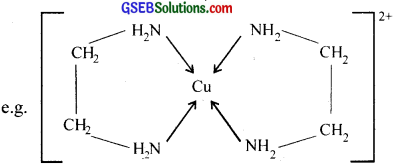The chelating ligands form more stable co-ordination compounds than the monodentate analogs.Question 27.
Discuss briefly giving an example in each case the role of coordination compounds in:

1. Biological systems
2. Medicinal chemistry
3. Analytical chemistiy
4. Extraction/metallurgy of metals.

The ability of metal ions to form complexes with a variety of molecular species with different physico-chemical properties is utilized in many ways. For example,

1. In analytical chemistry:

a. Multidentate ligand, EDTA (Ethylene Diarnine Tetra Acetic acid) forms highly stable complex with metal ions like Ca2+ and Mg2+. This fact is used to estimate the hardness of water by a simple titration method using EDTA.

b. A confirmatoiy test for the detection of copper (Il) involves the formation of a deep-blue coloured complex. [Cu(NH3)4]2+, on addition of ammonia solution to a solution ofcopper (II) salt.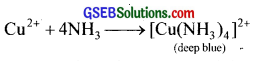c. The separation of group 1 precipitate (in qualitative inorganic analysis) of AgCl, Hg2Cl2, and PbCl2 involves the addition of aqueous ammonia solution lo the precipitate, when only silver chloride dissolves, due to the formation of the complex ion, [Ag(NH3)2]+.
AgCl + 2NH3 → [Ag(NH3)2]+ + Cl
Hg2Cl2 and PbCl2 do not form complex ions with NH3 and hence, do not dissolve.

d. A confirmatory test for nickel involves the addition ola solution ofdimethyl glyoxime, when a scarlet red coloured precipitate is formed, due to the formation ola chelate complex.

2. In extraction of metals:

a. Extraction of metals like Ag and Au is carried out by forming their soluble cyanide complexes.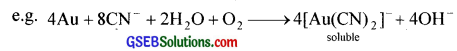The solution containing cyanide complex is then treated with zinc when gold is precipitated.
2[Au(CN)2] + Zn → [Zn(CN)4]2- + 2Au

b. Co-ordination compounds of silver and gold are used as the constituents of electroplating baths for the controlled delivery of Ag+ and Au+ ions, during clectrorefining of these metals.

c. The formation of volatile [Ni(CO)4] is used in the purification of nickel in Mond process.

3. Medicines:
Many medicines are complexes. Vitamin – B12 complex of cobalt is essential to prevent anaemia. The co-ordination compound cis-[PtCl2(NH3)2] commonly known as cisplatin is used in chemotherapy for the treatnierit of cancer. Complexing agents arc used for the removal of metal poisoning.

For example, D-penicillamine is used as a medicine to cure copper poisoning EDTA is used in the treatment of lead poisoning. A person who happens to be affected by atomic plant explosion may have imbibed a lot of radioactive materials like Pu. The best way to get rid of these metals is to treat the affected person with good chelating agents (Chelateherapy).

4. Photographs:
The unexposed AgBr in the film is dissolved with a solution of sodium thiosuiphate and the dissolution occurs due to the formation of a soluble silver thiosuiphato complex.
AgBr + 2Na2S2O3 → Na3[Ag(S2O3)2] + NaBr
This process is known as fixing.

5. Biological systems:
Chlorophyll isa coordination compound of magnesium (II) and it displays a catalytic role in the photosynthesis of glucose from CO2 and H2O. Haemoglohin is a coordination compound of iron (II) and it is responsible for oxygen transport. The metalloenzymes are nothing but coordination compounds. The enzymes like carboxy peptidase A and carbonic anhydrase are also coordination compounds.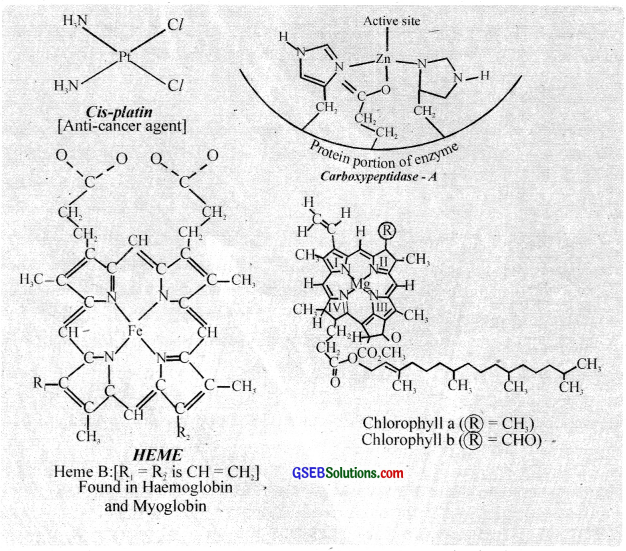6. Industrial processes:

a. Zeigler-Natta catalysts [(C2H5)3Al and TiCl4 are used as heterogeneous catalysts for the polymerisation of alkenes.

b. Wilkinsons catalyst [(Ph3P)3RhCl] is used as a catalyst for the hydrogenation of alkenes.

Question 28.
How many ions are produced from the complex Co(NH3)6]Cl2 in solution?

1. 6
2. 4
3. 3
4. 2

Since the coordination number of cobalt is 6, the complex is [Co(NH3)6]Cl2. It ionises to give [Co(NH3)6]2+ and 2Cl ions.
∴ Three ions are produced.Question 29.
Amongst the following ions which one has the highest magnetic moment value?

1. [Cr(H2O)6]3+
2. [Fe(H2O)6]2+
3. [Zn(H2O)6]2+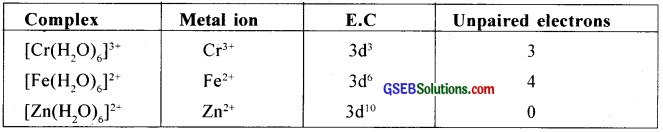As the number of unpaired electrons increases the magnetic moment increases.
∴ [Fe(H2O)6]3+ has the highest magnetic moment.

Question 30.
The oxidation number of cobalt in K[Co(CO)4 is

1. +1
2. +3
3. -1
4. -3

3. – 1

Question 31.
Amongst the following, the most stable complex is

1. [Fe(H2O)6]3+
2. [Fe(NH3)6]3+
3. [Fe(C2O4)3]3-
4. [FeCl6]3-

C2O2-4 is a bidentate chelating ligand and it forms chelate rings and hence is the most stable complex. Hence the answer (iii).Question 32.
What will be the correct order for the wavelengths of absorption in the visible region for the following?
[Ni(NO2)6]4-, [Ni(NH3)6]2+, [Ni(H2O)6]2+
1. The order of the ligand in the spectrochemical series:
H2O < NH3 < NO2

Hence the wavelength of the light observed will be in the order:
[Ni(H2O)6]2+ < [Ni(NH3)6]2+ < [Ni(NO2)6]4-
Thus, wavelengths absorbed (E = $$\frac{hc}{λ}$$) will be in the opposite order.

### GSEB Class 12 Chemistry Coordination Compounds Additional Important Questions and Answers

Question 1.
Werner’s co-ordination theory is useful for explaining the formation of co-ordination compounds?

(I) Write the postulates of Werner’s coordination theory.
(II) Write an example of a polydentate ligand.
(III) Write the formulae of the following co-ordination compounds.

a. hexamminecobalt (III) chloride
b. dichloridodiammineplatinum (II)

(I) In order to explain the bonding in co-ordination compounds, Alfred Werner (1893) proposed a theory known as Werner’s co-ordination theory. The important postulates of the theory are given below.

a. Metals possess two types of valencies – primary and secondary. The primary valency is ionisable while the secondary valency is non-ionisabie.

b. Every metal atom or ion has a fixed number of secondary valencies equal to its co-ordination number.

c. The primary valencies are satisfied by negative ions and the secondary valencies by negative or neutral groups (ligands).

d. The ligands satisfying the secondary valencies are always directed towards fixed positions in space giving a definite geometry to the complex. The primary valencies are non-directional.
For example, [Co(NH3)6]Cl3 [hexaamminecobalt (III) chloride] can be represented as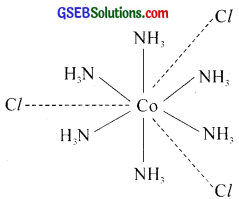Here three dotted lines indicate three primary valencies (oxidation state) of cobalt; while the six thick lines indicate its secondary valencies (co-ordination number).

(II) A polydentate ligand has several donor sites, e.g. Ethylenediammine tetra acetic acid

(III)
a. [Co(NH3)6Cl3
b. [Pt Cl2(NH3)2]Question 2.
Ramu found that when CuSO4 is mixed with NH3 a blue coloured solution is obtained?

1. Identify the compound formed?
2. Write the IUPAC name of the compound?
3. What do you understand by the terms co-ordination number and ligand?
4. Explain the structure of tetracarbonyl nickel with the help of valence bond theory?

1. When CuSO4 is mixed with ammonia, a blue coloured solution is obtained due to the formation of cuprammonium complex.
CuSO4 + 4NH3 → [Cu(NH3)4]SO4

2. Tetraamminecopper (II) sulphate.

3. The total number of ligands surrounding the central metal atom or ion in the co-ordination sphere is called the co-ordination number. For example, the co-ordination number of Cr3+ ion in [Cr(NH3)6]Cl3 is six.

An atom or group of atoms which binds to the central atom through the lone pair of electrons present in its donor atoms is called a ligand. Thus, a ligand may be a neutral molecule or ion having atleast one lone pair of electrons, which can co-ordinate to a metal.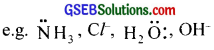4. Tetracarbonylnickel (0), Ni(CO)4]:
Here nickel is present in zero oxidation state. Ni has 3d84s2 electronic configuration. Since CO is a strong field ligand, the 4s electrons are forced to pair up against the Aufbau principle to make the 4s orbitais empty.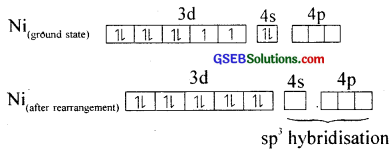The empty 4s and three 4p orbitais undergo sp3 hybridisation to form four equivalent hybrid orbitais which accept electron pairs from four CO and form Ni(CO)4.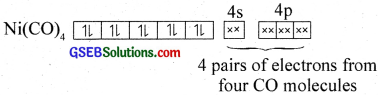Thus, nickel tetracarbonyl is a low spin (diamagnetic – no unpaired electron) outer orbital complex with tetrahedral geometry.

Question 3.
What is the co-ordination number? Give any two examples.
Co-ordination number: The number of ligands which are directly linked with central metal or metal ion by co-ordinate bonds, is the co-ordination number.
Example : (i) [CO(NH3)6]Cl3 co-ordination number of CO3+ ion is 6.
(ii) In [Ag(CN)2] co-ordination number of Ag is 2.Question 4.
Cisplatin is used for the treatment of cancer.

1. What is cisplatin?
2. Write the IUPAC name of the following coordination compounds
• K3[Fe(C2O4)]
• [Pt(NH3)6]Cl4
3. Using art example write about ionization isomerism shown by co-ordination compounds.
4. Explain with the help of electronic configuration why [Fe (CN)6]3- is paramagnetic.

1. Cis[PtCl2(NH3)2 is known as cisplatin.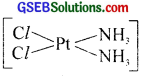2.
a. K3[Fe(C2O4)3] – potassiumtrioxalato ferrate (III)
b. [Pt (NH3)6]Cl4 – hexaammine platinum (IV) chloride.

3. Ionisation isomerism arises due to the interchange of groups between the co-ordination sphere of the metal ion and ions outside the co-ordination sphere. Therefore, the isomers can give different ions in their solutions.
e. g. Violet coloured [Co(NH3)5Br]SO4 is the ionisation isomer of red coloured [Co(NH3)3SO4]Br.

4. In [Fe(CN)]3-, iron is in +3 oxidation state and has 3d5 electronic configuration. In the presence of strong ligand CN~ ion, the d electrons are forced to pair leaving only one unpaired electron.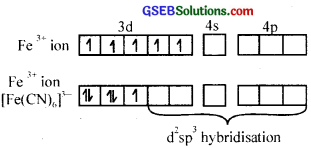Due to the presence of one unpaired electron, it should be slightly paramagnetic.

Question 5.
“Strength of the ligand can affect the magnetic property of the co-ordination complex”?

1. Can you agree with this statement?
2. Illustrate the statement by taking two complexes?

1. Yes.
2. i. Tetracyanonickelate (II) ion, [Ni (CN)4]2-
Here CN is a strong ligand. So electrons in 3d – orbitals are forced to pair, resulting in diamagnetism.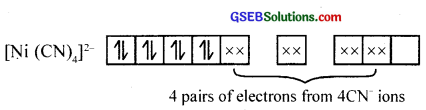2. Hexafluorocobaltate (III) ion, [CoF6]3-
Here F is a weak ligand. So pairing of 3d electrons will not take place. So unpaired elec¬trons are there and it gives paramagnetic behaviour.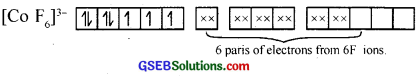Question 6.
Coordination compounds are used for the extraction of metals?

1. Write one application each of the coordination compounds in analytical chemistry and in the extraction of metals.
2. Write the formulae of,
• tetracyanonickelate (II)
• hexaamminecobalt (III) chloride
3. [CO(NH3)6]3+ is diamagnetic, whereas [CoF6]3- is paramagnetic. Why?

1. In the qualitative analysis of group II cations, the Cu2+ ions are detected by the formation of a deep blue coloured complex with ammonia.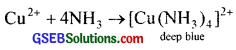Metals like Ag, Au etc. are extracted by converting them into their soluble cyanide complexes,
e.g. Ag2S + 4 NaCN → 2 Na [Ag(CN)2] + Na2S
2Na [Ag(CN)2] + Zn → Na2[Zn(CN)4 + 2 Ag

2.

• [Ni(CN)42-
• [Co(NH3)6]Cl3

3. F is a weak ligand and is unable to pair up the unpaired electrons of the 3d orbitals and NH3 is a strong field ligand and as it approaches the metal ion, the electrons must pair up. Therefore [CoF6]3- contains unpaired electrons and hence it is paramagnetic but [Co(NH3)6]3+ is not.

Question 7.
(a) Ramu found that [Pt(NH3)2Cl2 and [Pt(NH3)6]Cl4 will differ in their electrolytic conductances? Why?
(b) What are the important uses of coordination compounds?
(a) [Pt(NH3)2Cl2] will not ionise.
∴ Thus it will not produce ions in the solution. The electrolytic conductance of it will be almost negligible. On the other hand [Pt (NH3)6]Cl4 will ionise in solution to produce [Pt(NH3)6]4+ and four cl ions. Its conductance will be high as there will be 5 mole of ions in the solution.

(b) The ability of metal ions to form complexes with a variety of molecular species with different physico-chemical properties is utilized in many ways. For example.

(I) In analytical chemistry:

(a) Multidentate ligand, EDTA (Ethylene Diamine Tetra Acetic acid) forms highly stable complex with metal ions like Ca2+ and Mg2+. This fact is used to estimate the hardness of water by a simple titration method using EDTA.

(b) A confirmatory test for the detection of copper (II) involves the formation of a deep-blue coloured complex., [Cu(NH3)4]2+, on addition of ammonia solution to a solution of copper (II) salt.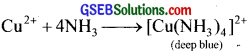(c) The separation of group 1 precipitate (in qualitative inorganic analysis) of AgCl, Hg2Cl2 and PbCl2 involves the addition of aqueous ammonia solution to the precipitate, when only silver chloride dissolves, due to the formation of the complex ion. [Ag(NH3)2]+
AgCl + 2NH3 → [Ag(NH3)2]+ + Cl
HgCl2 and PbCl2 do not form complex ions with NH3 and hence, do not dissolve.

(d) A confirmatory test for nickel involves the addition of a solution ofdimethyl glyoxime. when a scarlet red coloured precipitate is formed, due to the formation of a chelate complex.

(II) In extraction of metals:

(a) Extraction of metals like Ag and Au is carried oUt by forming their soluble cyanide complexes.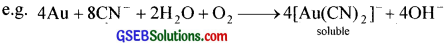The solution containing cyanide complex is then treated with zinc when gold is precipitated.
2[Au(CN)2] + Zn —* [Zn(CN)4]2 + 2 Auub>2] + Zn → [Zn(CN)4]2- + 2 Au

(b) Co-ordination compounds of silver and gold are used as the constituents of electroplating baths for the controlled delivery of Ag+ and Au+ ions, during electrorefining of these metals.

(c) The formation of volatile [Ni(CO)4] is used in the purification ofnickel in Mond process.

(III) Medicines:
Many medicines are complexes. Vitamin – B12 a complex of cobalt is essential to prevent anaemia. The co-ordination compound cis-[PtCl2(NH3)2] commonly known as cisplatin is used in chemotherapy for the treatment of cancer. Complexing agents are used fòr the removal of metal poisoning. For example, D-penicillaniine is used as a medicine to cure copper poisoning.

EDTA is used in the treatment of lead poisoning. A person who happens to be aflected by atomic plant explosion may have imbibed a lot of radioactive materials like Pu. The best way to get rid of these metals is to treat the affected person with good chelating agents (Chelate therapy).

(IV) Photography:
The unexposed AgBr in the film is dissolved with a solution of sodium thiosuiphate and the dissolution occurs due to the formation of a soluble silver thiosulphato complex.
AgBr + 2Na2S2O3 → Na3[Ag(S2O3)2] + NaBr
This process is known as fixing.

(V) Biological systems:
Chlorophyll ¡s a coordination compound of magnesium (II) and it displays a catalytic role in the photosynthesis of glucose from CO2 and H2O. Haemoglohin isa coordination compound of iron (II) and it is responsible for oxygen transport. The metalloenzymes are nothing but coordination compounds. The enzymes like carboxy peptidase A and carbonic anhydrase are also coordination compounds.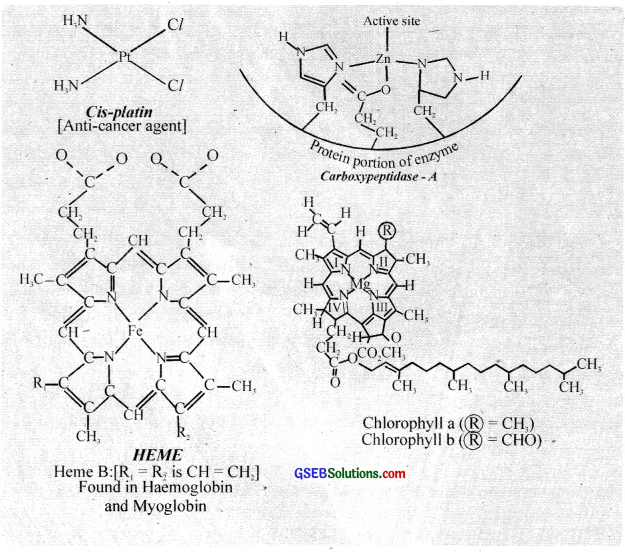(VI) Industrial processes:

• Zeigler-Natta catalysts [(C2H5)3Al and TiCl4) are used as heterogeneous catalysts for the
polymerisation of alkenes.
• Wilkinson’s catalyst [(Ph3P)3RhCl] is used as a catalyst for the hydrogenation of alkenes.Question 8.
Match the following